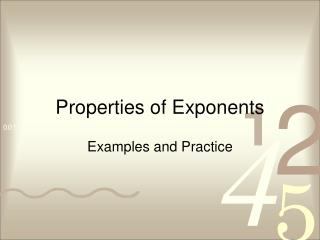Download PresentationProperties of Exponents

# Properties of Exponents

Download Presentation## Properties of Exponents

- - - - - - - - - - - - - - - - - - - - - - - - - - - E N D - - - - - - - - - - - - - - - - - - - - - - - - - - -
##### Presentation Transcript

1. Properties of Exponents Examples and Practice

2. Product of Powers Property • How many factors of x are in the product x3∙x2? • Write the product as a single power. • In general: 5 factors: x∙x∙x∙x∙x x∙x∙x∙x∙x = x5 xm∙xn= xm + n

3. Question #1 • Simplify the expression: a3a5 a. a15 b. a8 c. a2 d. 1/a2

4. Question #2 • Simplify the expression: (3m2)(2m4) a. 6m8 b. 5m6 c. 5m8 d. 6m6

5. Question #3 • Simplify the expression: (-2xy3)(5x4y2) a. -10x5y5 b. -10x4y5 c. 3x5y5 d. -10x4y6

6. Power of a Power Property • How many factors of x are in the expression (x3)2? • Write the product as a single power. • In general: 6 factors: x∙x∙x∙x∙x∙x (x∙x∙x)∙(x∙x∙x) = x6 (xm)n= xm∙n

7. Question #4 • Simplify the expression: (42)5 a. 47 b. 1610 c. 410 d. 167

8. Question #5 • Simplify the expression: (x3)4 a. x7 b. 2x7 c. x12 d. 2x12

9. Power of a Product Property • How many factors of x and y are in the expression (xy)2? • Simplify the expression. • In general: 2 factors of each: (x∙y)∙(x∙y) (x∙y)∙(x∙y)= x2y2 (x∙y)m= xmym

10. Question #6 • Simplify the expression: (b3c2)4 a. b7c6 b. b12c8 c. b7c8 d. 2b12c8

11. Question #7 • Simplify the expression: (-3a3b)2 a. 6a5b2 b. 9a5b2 c. -9a6b2 d. 9a6b2

12. Question #8 • Simplify the expression: (-3a3b)2(2ab) a. 36a7b3 b. 18a7b3 c. -6a7b3 d. -18a7b3

13. Quotient of Powers Property • Simplify the expression. • In general:

14. Question #9 • Simplify the expression: a. a5 b. a9 c. 1/a5 d. 1/a9

15. Question #10 • Simplify the expression: a. a5 b. 6 c. 1/a5 d. 1/6

16. Question #11 • Simplify the expression: a. -3a5 b. -16a5 c. -3a8 d. -16a8

17. Question #12 • Simplify the expression: a. b. c. d. 0.75a4b3

18. Power of a Quotient Property • Simplify the expression. • In general:

19. Question #13 • Simplify the expression: a. b. c. d. 0.2

20. Question #14 • Simplify the expression: a. b. c. d.

21. Zero Exponent Property • In general:

22. Question #15 • Simplify the expression: x0y2 a. b. xy2 c. y2 d.